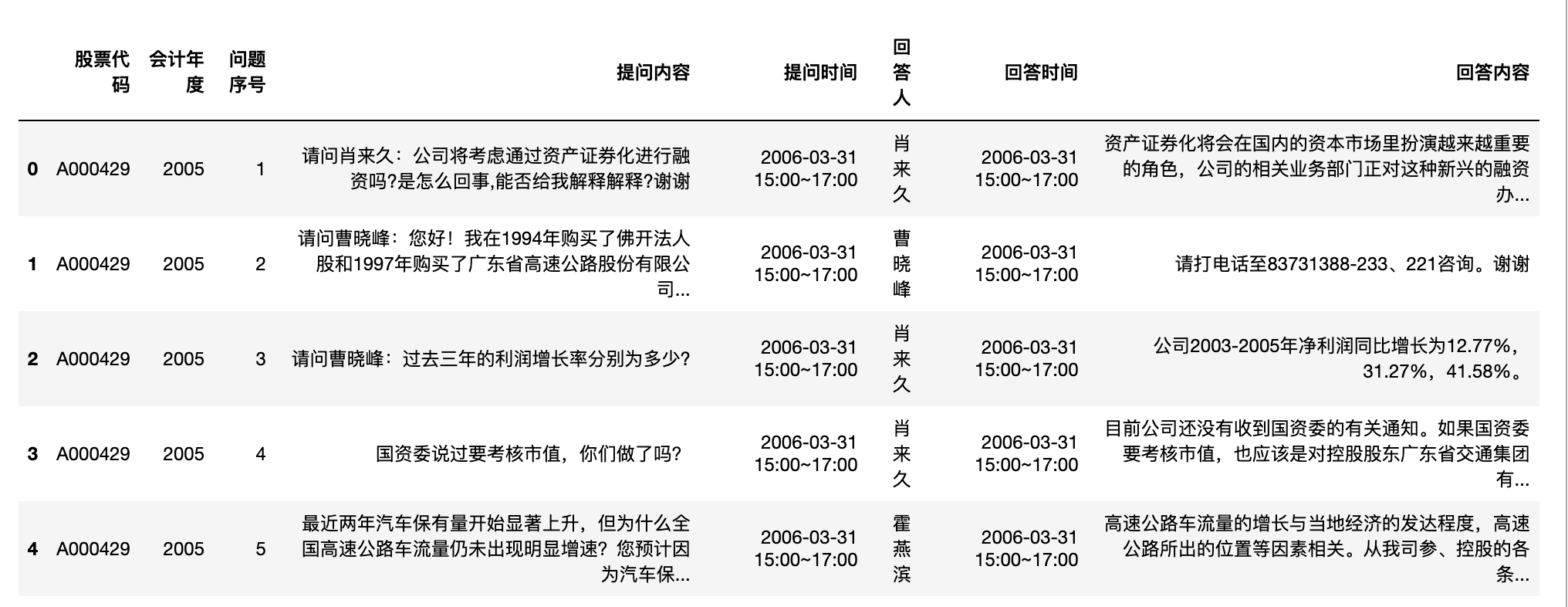• 公司沟通策略的研究：业绩说明会的数据可以帮助研究者深入了解公司如何与公众沟通其财务状况、业务策略和未来展望。这对于传播学、公关和企业战略研究领域都是宝贵的。
• 情感分析与市场反应：通过对业绩说明会中的语言和情感进行分析，研究者可以探索市场对公司信息披露的反应。这对于金融经济学和计量经济学的研究尤为重要。
• 公司治理与透明度：业绩说明会的频率、内容和与投资者的互动可以为研究者提供关于公司治理质量和透明度的线索。
• 预测模型的建立：这种数据集可以用于建立预测模型，预测公司的未来业绩、股价走势或其他相关指标。
• 行为金融学的研究：业绩说明会中的问题和答案可以为研究者提供关于投资者和分析师行为和心理的深入了解，从而深化我们对市场非理性行为的理解。
• 宏观经济指标的研究：通过对多家公司的业绩说明会数据进行汇总和分析，研究者可以获得宏观经济趋势和行业动态的宝贵见解。

## 数据集介绍#

上市公司业绩说明会问答数据

【年度】
2005-2023年

【字段】
- 股票代码
- 会计年度
- 问题序号
- 提问内容
- 提问时间
- 回答人
- 回答时间
- 回答内容

【数据量】
841876


## 导入数据#

import pandas as pd'数据集覆盖的年度: {start}~{end}'.format(start = df['会计年度'].min(),
end = df['会计年度'].max())

'数据集覆盖的年度: 2005~2023'


#数据量
len(df)

841876


#字段包括
df.columns

Index(['股票代码', '会计年度', '问题序号', '提问内容', '提问时间', '回答人', '回答时间', '回答内容'], dtype='object')


import matplotlib.pyplot as plt
import matplotlib
import matplotlib_inline
matplotlib_inline.backend_inline.set_matplotlib_formats('png', 'svg')
#pip3 install scienceplots
import scienceplots
import platform
plt.style.use(['science', 'no-latex', 'cjk-sc-font'])
system = platform.system()  # 获取操作系统类型

if system == 'Windows':
font = {'family': 'SimHei'}
elif system == 'Darwin':
font = {'family': 'Arial Unicode MS'}
else:
font = {'family': 'sans-serif'}
matplotlib.rc('font', **font)  # 设置全局字体

plt.figure(figsize=(10, 6))


#年份变化(业绩说明会数量)
df['会计年度'].value_counts().sort_index()

会计年度
2005     4042
2006    10051
2007    18906
2008    31782
2009    35802
2010    47141
2011    69439
2012    73231
2013    80456
2014    80690
2015    62764
2016    61820
2017    52543
2018    44279
2019    42009
2020    37026
2021    53898
2022    35917
2023       80
Name: count, dtype: int64


df['会计年度'].value_counts().sort_index().plot(kind='bar')
plt.xticks(rotation=0)
plt.xlabel('会计年度')
plt.ylabel('业绩说明会数量')
plt.title('业绩说明会数量年份变化')
plt.show()df.groupby(['会计年度', '股票代码'])['问题序号'].count().groupby('会计年度').mean().plot()

plt.xticks(rotation=0)
plt.xlabel('会计年度')
plt.ylabel('年度问答次数')
plt.title('业绩说明会平均问答次数年份变化')
plt.show()